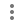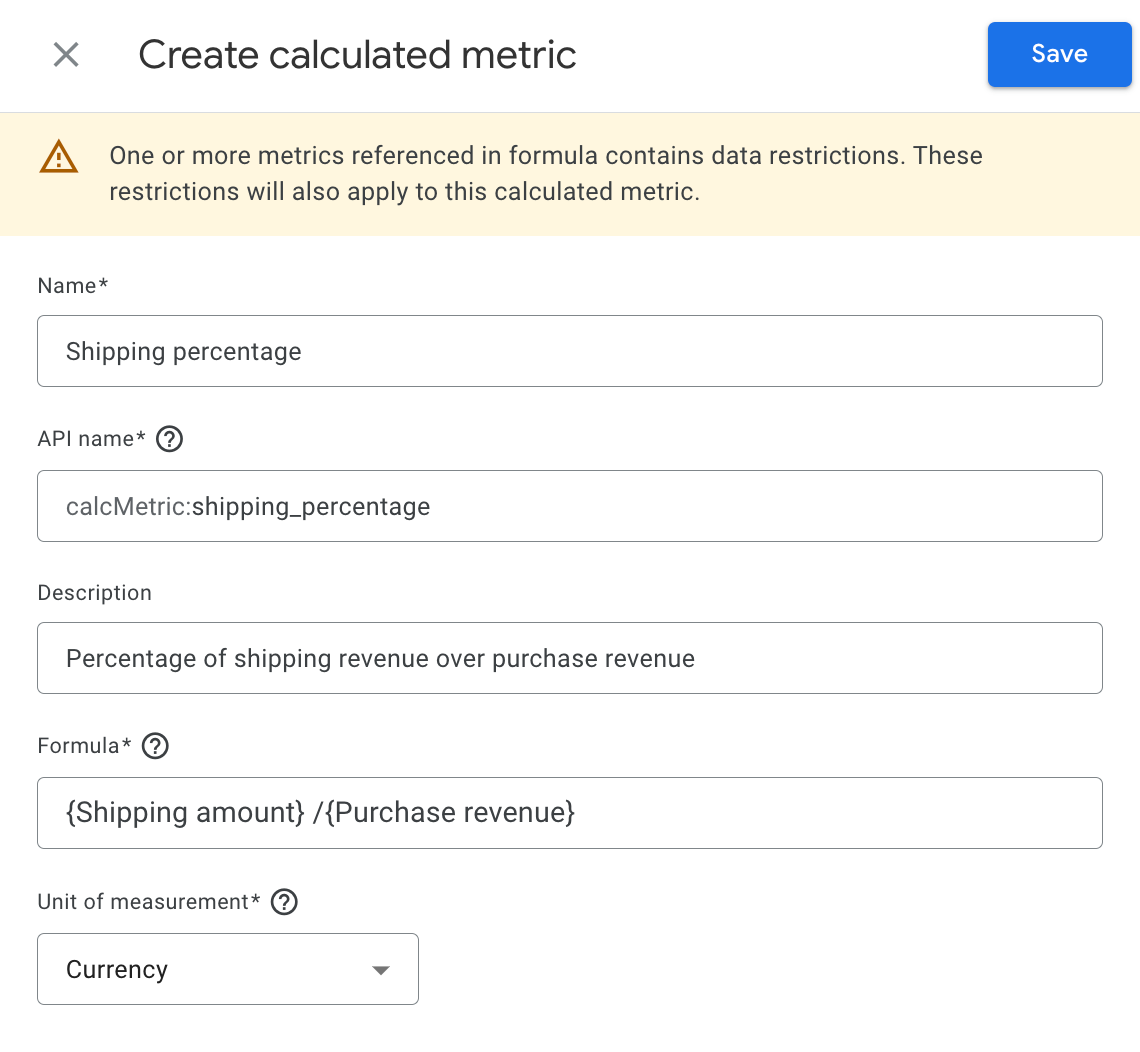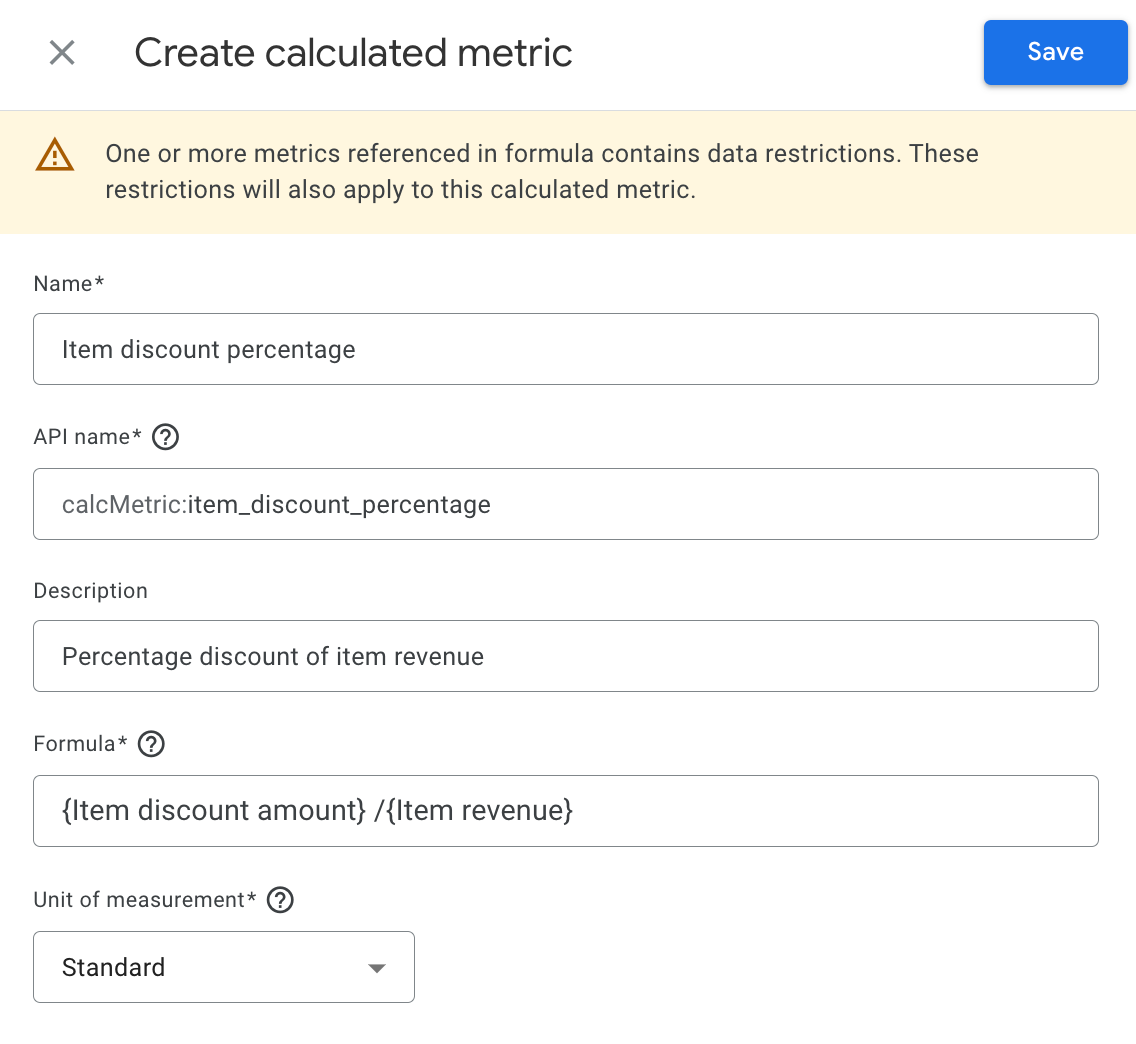# [GA4] Calculated metrics

Create your own metrics using existing and custom metrics to define a formula for a new metric

Calculated metrics are metrics that combine one or more existing or custom metrics within a mathematical formula to produce a new and potentially more valuable metric. For example, an Item margin metric could be created using the Item price standard metric and the Item COGS custom metric.

With calculated metrics, you can adjust any metric to fit your business needs or logic. You can weight or discount existing metrics and create new metrics by combining standard and/or custom metrics.

These new metrics are typically more actionable than the original metrics from which they're created, as they contain the additional business logic that enables direct decision making and action in Google Analytics.

You can access calculated metrics in reports, explorations, and the Google Analytics Data API.

## Prerequisites

You must be an Administrator or Editor to create a calculated metric.

## Limits & caveats

You can create up to 5 calculated metrics per standard property and 50 calculated metrics per 360 property.

You can't reference a calculated metric in the formula for another calculated metric. You can only use predefined metrics and custom metrics in the formula for a calculated metric.

## Create a calculated metric

1. Click Custom definitions.
2. In the Calculated metrics tab, click Create calculated metric.
3. Complete the following fields to define the calculated metric. See examples to get started.
Field Description
Name Enter a name for the calculated metric. The name you choose appears in the metric selectors for reports. The name must be unique across all other calculated and custom metrics. You can change the name later.
API name

Google populates the API name based on the value in the Name field. You can change the populated name during creation, but you can't change the name once you create the calculated metric.

The API name must be unique across all other calculated and custom metrics. However, if you archive the calculated metric, you can use the API name for a different calculated metric.

The API name can contain alphanumeric characters and underscore only. Special characters, symbols, and spaces are not allowed.

Description Explain the calculated metric to other users of the Google Analytics property, who may not be aware of how the calculated metric is computed. The description is available in reports when hovering over the metric.
Formula

Start typing to see a list of predefined metrics you can use to define the formula. You can also enter numbers and decimals. Metric names must be surrounded by single curly brackets {}. Formulas are limited to 1024 characters.

You can enter operators between metrics and numbers to define the formula. Accepted operators include:

- (minus or negative)
* (multiplication)
/ (division)
() (parenthesis)

Unit of measurement Select your desired unit of measurement, which determines how you calculated metric appears in reports.
4. Click Save to create the calculated metric.

## Edit a calculated metric

1. Click Custom definitions.
2. In the Calculated metrics tab, click Create calculated metric.
3. To the right of the calculated metric in the table, click More [] > Edit.
4. Edit the calculated metric. You can change the name, description, formula, and unit of measurement.
5. Click Save.

## Copy a calculated metric

1. Click Custom definitions.
2. In the Calculated metrics tab, click Create calculated metric.
3. To the right of the calculated metric in the table, click More [] > Copy.
4. Edit the copied version of the calculated metric.
5. Click Save.

## Use cases

### Example 1: Shipping revenue as % of purchase revenue• Name: Shipping percentage
• API name: (automatically populated)
• Formula: {Shipping amount} / {Purchase revenue}
• Unit of measurement: Currency

### Example 2: Item discount percentage• Name: Item discount percentage
• API name: (automatically populated)
• Formula: {Item discount amount} / {Item revenue}
• Unit of measurement: Standard